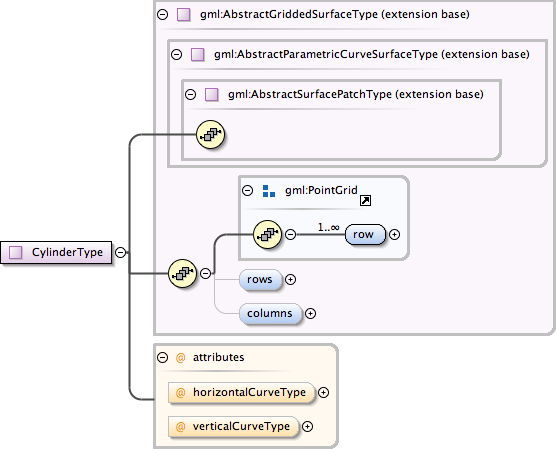### Showing:

 Annotations Attributes Diagrams Facets Properties Source Used by
Complex Type gml:CylinderType
Namespace http://www.opengis.net/gml
Annotations
 ```A cylinder is a gridded surface given as a family of circles whose positions vary along a set of parallel lines, keeping the cross sectional horizontal curves of a constant shape. NOTE! Given the same working assumptions as in the previous note, a Cylinder can be given by two circles, giving us the control points of the form ((P1, P2, P3),(P4, P5, P6)).```
DiagramType extension of gml:AbstractGriddedSurfaceType
Type hierarchy
Used by
 Element gml:Cylinder
Model gml:row+ , gml:rows{0,1} , gml:columns{0,1}
Children gml:columns, gml:row, gml:rows
Attributes
QName Type Fixed Default Use Annotation
horizontalCurveType gml:CurveInterpolationType circularArc3Points optional
verticalCurveType gml:CurveInterpolationType linear optional
Source
 ``` A cylinder is a gridded surface given as a family of circles whose positions vary along a set of parallel lines, keeping the cross sectional horizontal curves of a constant shape. NOTE! Given the same working assumptions as in the previous note, a Cylinder can be given by two circles, giving us the control points of the form ((P1, P2, P3),(P4, P5, P6)). ```
Schema location http://schemas.opengis.net/gml/3.1.1/base/geometryPrimitives.xsd
Attribute gml:CylinderType / @horizontalCurveType
Namespace No namespace
Type gml:CurveInterpolationType
Properties
 fixed: circularArc3Points
Facets
 enumeration linear enumeration geodesic enumeration circularArc3Points enumeration circularArc2PointWithBulge enumeration circularArcCenterPointWithRadius enumeration elliptical enumeration clothoid enumeration conic enumeration polynomialSpline enumeration cubicSpline enumeration rationalSpline
Used by
 Complex Type gml:CylinderType
Source
 ``
Schema location http://schemas.opengis.net/gml/3.1.1/base/geometryPrimitives.xsd
Attribute gml:CylinderType / @verticalCurveType
Namespace No namespace
Type gml:CurveInterpolationType
Properties
 fixed: linear
Facets
 enumeration linear enumeration geodesic enumeration circularArc3Points enumeration circularArc2PointWithBulge enumeration circularArcCenterPointWithRadius enumeration elliptical enumeration clothoid enumeration conic enumeration polynomialSpline enumeration cubicSpline enumeration rationalSpline
Used by
 Complex Type gml:CylinderType
Source
 ``
Schema location http://schemas.opengis.net/gml/3.1.1/base/geometryPrimitives.xsd